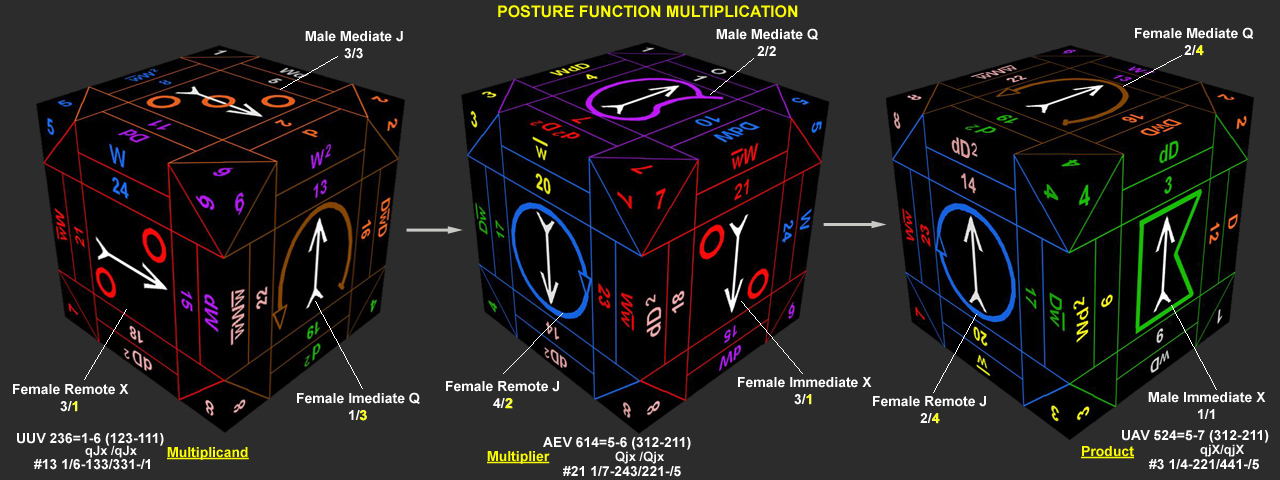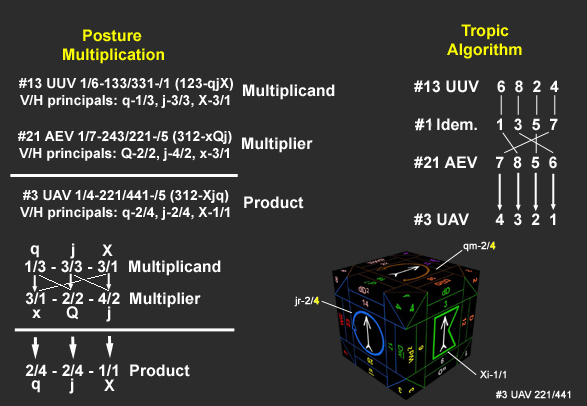Let’s examine the postures in more detail with the multiplication of full posture functions from one paraperm with another. Take #13,Upright U Vex=133/331=(123-212) as the multiplicand; #21,Averse E Vex=243/221=(312-212) as the multiplier.

Since #13 has its principal perm as 123 and #21 has 312, when multiplying the two, the principals in the multiplier must correspond to their same i,m,r principals above in the multiplicand. (#13)123=qJx and (#21)312=xQj. The i of #13 goes with the m of #21, but the product is put below in the i position since the principal order is governed by that of the *multiplicand* (#13), not its multiplier. Then the m of #13 goes with the r of #21 and the product is put below in the m place (again based upon the multiplicand’s principal perm). Finally, the r of #13 finds the i of #30 and the product is in the r position below. For the three principal products we have: i=2/2x1/3(Q)=2/4, m=4/2x3/3(J)=2/4 & r=3/1x3/1(X)=1/1. The product postures are then: 221/441. The whole product is: #3 UAV=1/4-221/441-/5.This multiplication may also be verified using the tropic algorithm, e.g.,#13(6824)x#21(7856)=#3(4321).The next section will continue further with posture function multiplication.

BACK